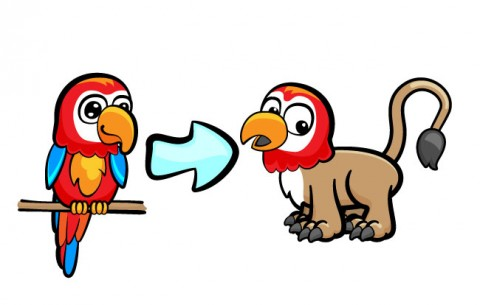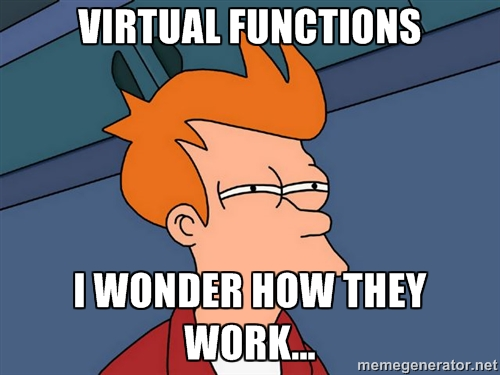# Introduction to C++ for iOS Developers: Part 2

In part 2 of this introduction to C++ for iOS developers, you will learn about polymorphism, virtual functions, templates, the STL, and more. By Matt Galloway.

Leave a rating/review
Save for later
Share

## Introduction to C++ for iOS Developers: Part 2

45 mins

Hello, C++!Welcome back to second part of the Introduction to C++ for iOS Developers series!

In the first part of this series, you learned about classes and memory management.

In this second second and final part of the series, you’ll dive deeper into classes and look at some more interesting features. You’ll see what a “template” is and then have a look at the Standard Template Library.

Finally, you’ll round it all off with learning about Objective-C++, which is a way to mix C++ into Objective-C.

## Polymorphism

Polymorphism is not a parrot that changes shape, contrary to what it might sound like!Put simply, polymorphism is the notion of overriding a function in a subclass. In Objective-C you probably have done this many times, for example when subclassing `UIViewController` and overriding `viewDidLoad`.

In C++, polymorphism goes quite a bit further than Objective-C. So stick with me while I explain this powerful feature.

To start with, here is an example of overriding a member function in a class:

```class Foo {
public:
int value() { return 5; }
};

class Bar : public Foo {
public:
int value() { return 10; }
};
```

But look at what happens if you do the following:

```Bar *b = new Bar();
Foo *f = (Foo*)b;
printf(“%i”, f->value());
// Output = 5
```

Wow — that’s probably not the output you expected! I suspect you thought it would output 10, right? This is where C++ differs drastically from Objective-C.

In Objective-C, it doesn’t matter if you cast a subclass pointer to its base class pointer. When you send a message (i.e. call a method) to any object, it is the runtime which looks up the class of the object and calls the most derived method. Therefore in this scenario in Objective-C, the subclass method on `Bar` is called.

This highlights the compile-time versus run-time difference that I mentioned in the first half of this series.

When the compiler encounters the call to `value()` in the above example, its job is to work out what function needs to be called. Since the type of `f` is a pointer to a `Foo`, it emits code to jump to `Foo::value()`. The compiler knows nothing about the fact that `f` is actually a pointer to a `Bar`.

In this simple example you could be forgiven for thinking that the compiler could reason that `f` is a pointer to a `Bar`. But consider what would happen if `f` were actually the input to a function. In that case the compiler could have no way of knowing that it’s actually a pointer to a class derived from `Foo`.

### Static Binding and Dynamic Binding

The above example illustrates perfectly the crucial difference between C++ and Objective-C; static versus dynamic binding. The behaviour seen above is an example of static binding. The compiler is responsible for resolving which function to call, and that behaviour is therefore baked in to the binary after compilation. There is no ability to change this behaviour at runtime.

This is in contrast to method calling in Objective-C, which is an example of dynamic binding. The runtime itself is responsible to decide which function should be called.

Dynamic binding is what makes Objective-C so very powerful. You may already be aware that it’s possible to add methods to a class at runtime, or swap method implementations. This could never be done in a statically bound language, where the calling behaviour is baked in at compile time.

But wait — there’s more to it than that in C++! While C++ is generally statically bound, there are mechanisms available to use dynamic binding; these are known as “virtual functions”.

### Virtual Functions and the Virtual Table

Virtual functions provide a mechanism for dynamic binding. It defers the choice of which function is called until runtime through the use of a lookup table — one for each class. However, this does introduce a slight overhead cost at runtime when compared to static binding. The table lookup needs to happen in addition to calling the function. With static binding, only the calling of the function needs to be performed.

Using virtual functions is as simple as adding the “virtual” keyword to the function in question. Taking the previous example and using virtual functions instead would look like the following:

```class Foo {
public:
virtual int value() { return 5; }
};

class Bar : public Foo {
public:
virtual int value() { return 10; }
};
```

Now consider what happens when you run the same code as before:

```Bar *b = new Bar();
Foo *f = (Foo*)b;
printf(“%i”, f->value());
// Output = 10
```

That’s better! That’s the output you presumably expected earlier, right? So dynamic binding can be done in C++, but you need to decide whether you want static or dynamic binding depending on the scenario you face.

This type of flexibility is commonplace in C++; it’s what makes C++ a multi-paradigm language. Objective-C largely forces you into strict patterns, especially if you are using the Cocoa framework. C++, on the other hand, leaves a lot of decisions up to the developer.

It’s now time to get dirty and look at how virtual functions work.## The Inner Workings of Virtual Functions

Before you can understand how virtual functions work, you need to understand how non-virtual functions work. Consider the following code:

```MyClass a;
a.foo();
```

If `foo()` is non-virtual, then the compiler will convert this into code that jumps directly to the `foo()` function of `MyClass`.

But remember, this is where the problem with non-virtual functions lies. Recall from the previous example that if the class is polymorphic then the compiler can’t know the full type of the variable, and therefore can’t know which function it should jump to. There needs to be a way to lookup the correct function at runtime.

To accomplish this lookup, virtual functions make use of a concept known as the virtual table or v-table; this is a lookup table that maps functions to their implementations and each class has access to one. When the compiler sees a virtual function being called, it emits code that retrieves the object’s v-table and looks up the correct function.

Look back at the example from above to see how this works:

```class Foo {
public:
virtual int value() { return 5; }
};

class Bar : public Foo {
public:
virtual int value() { return 10; }
};

Bar *b = new Bar();
Foo *f = (Foo*)b;
printf(“%i”, f->value());
// Output = 10
```

When you create `b`, an instance of `Bar`, its v-table will be the v-table for Bar. When this variable is cast to a Foo pointer, it doesn’t alter the contents of the object. The v-table is still the v-table for Bar, not Foo. Therefore when the v-table is looked up for the call to `value()`, the result is `Bar::value()` which will be called.Matt Galloway

Author